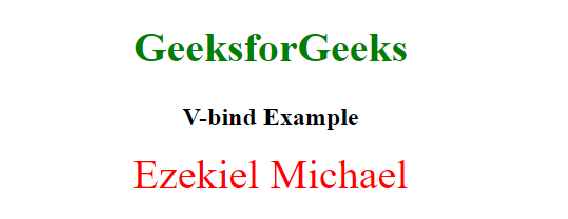# Difference between v-bind and v-model in Vue.js

• Last Updated : 11 Apr, 2022

This article covers the difference between v-model and v-bind in Vue.js. The following steps are used to explain their differences.

The v-model is a two-way binding which means if you change the input value, the bound data will be changed. The v-model directive is used to create two-way data bindings on form input, textarea, and select elements.

Example:

## HTML

 `` `<``html` `lang``=``"en"``>`   `<``head``>` `    ``<``meta` `charset``=``"UTF-8"``>` `    ``<``meta` `http-equiv``=``"X-UA-Compatible"` `content``=``"IE=edge"``>` `    ``<``meta` `name``=``"viewport"` `content``=` `        ``"width=device-width, initial-scale=1.0"``>` `    ``<``script` `src``=``"https://cdn.jsdelivr.net/npm/vue@2"``>` `    ``<``title``>Difference between v-model and v-bind` `    ``<``style``>` `        ``.container {` `            ``display: flex;` `            ``justify-content: center;` `            ``align-items: center;`   `        ``}`   `        ``p {` `            ``padding-left: 20px;` `        ``}`   `        ``h1,h2 {` `            ``text-align: center;` `        ``}` `        ``h1{` `            ``color: green;` `            ``font-size: 40px;` `        ``}` `    ``` ``   `<``body``>` `    ``<``section` `id``=``"app-vue"``>` `        ``<``h1``>GeeksforGeeks` `        ``<``h2``>V-Model Example` `        ``<``div` `class``=``"container"``>` `            ``<``input` `type``=``'text'` `v-model``=``'Message'` `/>` `            `  `<``p``> Message: {{ Message}} `     `        ``` `    ```   `    ``<``script``>` `        ``new Vue({` `            ``el: '#app-vue',` `            ``data() {` `                ``return {` `                    ``Message: ''` `                ``}` `            ``}` `        ``});` `    ``` ``   ``

Output: This example means that if our data changes, our input too will change, and if our input changes, our data changes too.The v-bind is called one-way binding which means it binds our data one way. It can also be used to bind HTML attributes. This example shows a one-way data-binding using our style element using v-bind.

Example:

## HTML

 `` `<``html` `lang``=``"en"``>`   `<``head``>` `    ``<``meta` `charset``=``"UTF-8"``>` `    ``<``meta` `http-equiv``=``"X-UA-Compatible"` `content``=``"IE=edge"``>` `    ``<``meta` `name``=``"viewport"` `content``=` `        ``"width=device-width, initial-scale=1.0"``>` `    ``<``script` `src``=``"https://cdn.jsdelivr.net/npm/vue@2"``>` `    ``<``title``>Difference between v-model and v-bind` `    ``<``style``>` `        ``.container {` `            ``display: flex;` `            ``justify-content: center;` `            ``align-items: center;` `        ``}     `   `        ``h1,` `        ``h2 {` `            ``text-align: center;` `        ``}`   `        ``h1 {` `            ``color: green;` `            ``font-size: 40px;` `        ``}` `    ``` ``   `<``body``>` `    ``<``section` `id``=``"app-vue"``>` `        ``<``h1``>GeeksforGeeks` `        ``<``h2``>V-bind Example` `        ``<``div` `v-bind:style``=``"headingText"``>` `          ``Ezekiel Michael` `       ``` `    ```   `    ``<``script``>` `        ``new Vue({` `            ``el: '#app-vue',` `            ``data: {` `                ``headingText: {` `                    ``color: 'red',` `                    ``fontSize: '40px',` `                    ``textAlign:'center'` `                ``}` `            ``}` `        ``});` `    ``` ``   ``

Output:Below are differences between v-model and v-bind

My Personal Notes arrow_drop_up
Recommended Articles
Page :